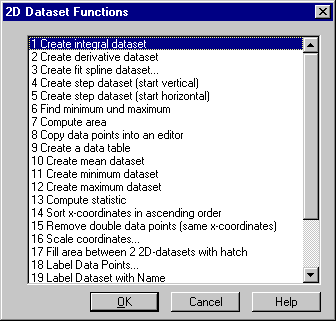# Data=>More Data Functions (2D)¶

In order for this command to be activated, a 2D dataset must be selected.Create integral dataset
Creates a integral dataset from the selected dataset. (see Data=>Integral).
Create derivative dataset
Creates a derivative dataset from the selected dataset (see Data=>Derivative).
Create fit spline dataset
Creates a spline dataset from the selected dataset (see Data=>Create fit spline dataset).
Create step dataset (start vertical)
Creates a new dataset with a step curve starting with a vertical line (see Data=>Step dataset vertical).
Create step dataset (start horizontal)
Creates a new dataset with a step curve starting with a horizontal line (see Data=>Step dataset horizontal).
Find minimum and maximum
Searches for the selected dataset’s absolute minimum and maximum values (see Data=>Find minimum and maximum).
Compute Area
Calculates the surface area of the selected dataset (see Data=>Compute area).
Create a data table
Creates a data table for the selected dataset as an Excel Object (see Data=>Create a data table).
Create a mean dataset
Creates a dataset with 2 points in which the y-coordinates of both points are the arithmetic mean of the y-coordinates of the selected dataset (see Data=>Create a mean dataset).
Create a minimum dataset
Creates a dataset with 2 points in which the y-coordinates of both points are the same as the minimum of the dataset’s y-coordinates (see Data=>Create a minimum dataset).
Create a maximum dataset
Creates a dataset with 2 points in which the y-coordinates of both points are the same as the maximum of the dataset’s y-coordinates (see Data=>Create a maximum dataset).
Compute statistic
Calculates some statistic values (see Data=>Compute statistic (2D)).
Sort x-coordinates in ascending order
Sorts the x-coordinates in ascending order (see Data=>Sort x-coordinates (2D)).
Remove double data points (same x-coordinates)
Removes double data points from the selected dataset (see Data=>Remove double data points).
Scale coordinates
Runs a linear scaling of the x- and y-coordinates of the selected dataset (see Data=>Scale coordinates (2D)).
Fill area between 2 X/Y-Datasets with hatch
This function creates a new dataset from 2 selected datasets. (see Data=>Fill Area (2D)).
Label Data Points
The function labels the data points with its y-values (see Data=>Label Data Points).
Label Dataset with Name
Lables the curve with its name (see Data=>Label Dataset with Name).
Create Data Boundary
Calculates the upper or lower boundary (see Data=>Create Data Boundary).
Reduce Signal Noise
Reduces noise by calculating the moving avarage (see Data=>Reduce Signal Noise).
Extract Data Subset
(see Data=>Extract Data Subset).
Compute Linear Correlation Coefficient
(see Data=>Compute Linear Correlation Coefficient).
Create Norm Dataset
(see Data=>Create Norm Dataset).
2D-Interpolation
This function can apply operations like add, sum, mean, etc. for 2D datasets which have monotone increasing x-coordinates but not necessarily with identical x-coordinates. (see Data=>Linear Interpolation):
Polynom Fit Functions
Calculates a polynom fit function for the selected dataset (see Data=>Polynom Fit Functions).
Other Fit Functions
Calculates a fit function for the selected dataset (see Data=>Fit Functions).
Compound Counting
See Data=>3D Classification of a XYZ-Dataset.

Comment

The functions for 2D datasets are written in UniScript. The source code can be found in the file `rs_xy.ic`.